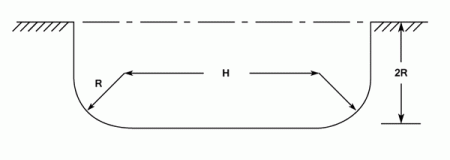•http://facebook.com/
•https://www.google.com/accounts/o8/id
•https://me.yahoo.com

# Uniform Flow

Introduces the Chezy and Manning formulae for open channel flow

## Introduction

Uniform Flow occurs in long inclined channels of uniform cross section when the terminal velocity is reached.

This occurs when the loss of potential energy equals the work done against the channel surface friction. In this condition the water surface is parallel to the bed of the channel.

## The Chezy Equation

The volumetric flow rate in fluid dynamics and hydrometry is the volume of fluid which passes through a given surface per unit time in SI units, or cubic feet per second. It is usually represented by the symbol .

Key facts
• Chezy Equation:

The Chezy Equation is analogous to the Darcy Equation used in the flow of liquid through pipes. Chezy states that: or:

where:
• is the velocity of flow;
• is the Chezy constant;
• is the slope of the channel or the slope of the hydraulic gradient;
• is the area of flow;
• is the wetted perimeter of the channel;
• is the hydraulic mean depth which equals .

### A Comparison Between The Chezy And Darcy Equations

Darcy states that:

where

This can be written as:

Thus the Darcy and Chezy equations are basically the same and

### The Chezy Constant C

The Chezy constant varies with the nature of the channel walls and with the Hydraulic mean depth. There are a number of Empirical equations which seek to eliminate the variations of with . Amongst these the Manning equation

### The Manning Equation

Key facts:
• Manning's formula
Manning substituted for the C of the Chezy equation. Hence:

This can be re-written by replacing the as follows:

In this form
• is a conversion constant and equals 1.486 in the Imperial system and 1.0 in SI units.
• is the Manning Coefficient and is independent of units. It depends only on the nature of the channel surface and varies from 0.01 (smooth) to 0.035 (rough)

## The Conditions For The Most Economic Channel Section

Three cross sectional areas will be considered .

### Rectangular Section

The maximum discharge (or maximum velocity) for a given area will occur when the wetted area, , is a minimum. For a unit length:

and .
Differentiating and equating to zero for the minimum wetted area.

i.e.

Thus for a maximum velocity of flow, or a maximum rate of flow,

### A Trapezoidal Channel

The maximum Flow or the maximum velocity of flow for a given area and slope occurs when the wetted area is a minimum. It can be shown that this happens when the sloping sides and the base are tangential to a semi-circle described on the water surface.

But since

Hydraulic Mean Depth ,

Using either the Chezy or Manning formulae, the maximum velocity for a given radius will occur when is a maximum.

i.e. When:

This occurs when:
i.e.
And the maximum depth of water is:
where is the diameter

Using the Chezy equation the maximum flow, will occur when is a maximum.

i.e. When:

This will occur when:

i.e.
And the maximum depth at the centre is:
where is the diameter of the channel

Or using the Manning Equation will be a maximum when is a maximum.

i.e. When:
From which:
The maximum depth at the centre is:
where is the diameter of the channel

Example:
[imperial]
##### Example - Uniform channel flow
Problem
Water flows down a uniform channel with a slope of . The cross section shown in the diagram.

If the channel is to convey 600 Cusec under the condition of maximum discharge for a given area find the values of and . the Chezy constant is 120.Workings
Using the Chezy Equation:

From the above equation it can be seen that for a given cross sectional area the flow will be maximum when the wetted area, is a minimum.

From Equation (1)

Substituting in equation(2)

Thus:

Differentiating and equating to zero for minimum value of :

Substitute from
Thus:
The Hydraulic Mean Depth
But:
Solution
From which:
And